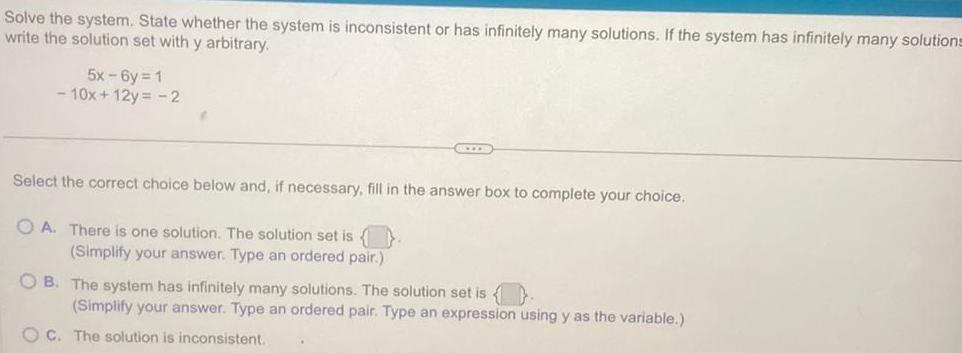Question:

# Solve the system State whether the system is inconsistent or

Last updated: 11/21/2023Solve the system State whether the system is inconsistent or has infinitely many solutions If the system has infinitely many solutions write the solution set with y arbitrary 5x 6y 1 10x 12y 2 Select the correct choice below and if necessary fill in the answer box to complete your choice OA There is one solution The solution set is Simplify your answer Type an ordered pair OB The system has infinitely many solutions The solution set is Simplify your answer Type an ordered pair Type an expression using y as the variable OC The solution is inconsistent